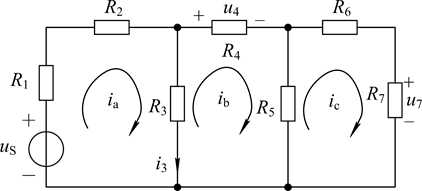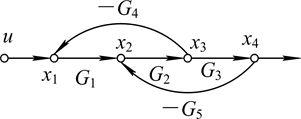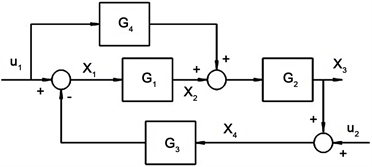﻿ 工科线性代数必需的三项改革—介绍《实用大众线性代数(MATLAB版)》教材及其慕课 Three Necessary Reforms in Linear Algebra for Engineering—Introduction to Book “Applied Linear Algebra with MATLAB”

Vol. 07  No. 09 ( 2018 ), Article ID: 26805 , 7 pages
10.12677/AAM.2018.79135

Three Necessary Reforms in Linear Algebra for Engineering

—Introduction to Book “Applied Linear Algebra with MATLAB”

Huaichen Chen1, Wei Yang2

1School of Electronics Engineering, Xidian University, Xi’an Shaanxi

2School of Physics and Optoelectronic Engineering, Xidian University, Xi’an ShaanxiReceived: Aug. 23rd, 2018; accepted: Sep. 6th, 2018; published: Sep. 13th, 2018ABSTRACT

Using reverse thinking method, dozens of engineering linear algebra problems were solved. We found that the biggest obstacle is the complexity of calculation, so computerization is the first key point. Showing innovative solutions using computer surpassing the old ones in engineering courses is the second point. The third is the deletion of useless mathematics theory. According to these principles, the textbook of “Applied Popular Linear Algebra with MATLAB” and its MOOC was launched.

Keywords:Linear Algebra, Determinant, Matrix Operation, Computerized Solution, MATLAB

—介绍《实用大众线性代数(MATLAB版)》教材  及其慕课

1西安电子科技大学，电子工程学院，陕西 西安

2西安电子科技大学，物理与光电工程学院，陕西 西安1. 引言

2. 线性代数能成为工科的数学工具吗？答案是：必须“现代化”

 ，则 ${A}^{-1}=\frac{1}{\mathrm{det}\left(A\right)}\left[\begin{array}{ccc}ek-fh& ch-bk& bf-ce\\ dk-fg& ak-cg& cd-af\\ dh-eg& bg-ah& ae-bd\end{array}\right]$

1950年代计算机的发明改变了这种局面。1970年代，线性代数软件包LINPACK开发成功，并诞生了MATLAB等矩阵软件工具，求一个几十阶方阵的逆只要几秒钟。工程界看到了它的价值，从那时起，世界各国都把线性代数引进了教学计划中，并成为必修的基础课。因为大学本科中许多课程都包含了线性代数方程组，工科希望能用新的方法来解。没想到这些线代老师带来的仍然是古典的代数方法，没有把最新的计算机解题技术带来，那当然使工科师生大失所望。

3. 线性代数能成为工科各课的基础吗？答案是：能，但必须“实用化”

$\begin{array}{l}\begin{array}{l}-2{c}_{1}+0-5{c}_{3}=1\\ 5{c}_{1}+{c}_{2}-{c}_{3}=1\\ 0-2{c}_{2}-{c}_{3}=1\end{array}\right\}⇒\left[\begin{array}{ccc}-2& 0& -5\\ 5& 1& -1\\ 0& -2& -1\end{array}\right]\left[\begin{array}{l}{c}_{1}\\ {c}_{2}\\ {c}_{3}\end{array}\right]=\left[\begin{array}{l}1\\ 1\\ 1\end{array}\right]\\ ⇒Ac=B⇒c=inv\left(A\right)*B\end{array}$

A = [−2, 0, −5; 5, 1, −1; 0, −2, −1]; B = [1; 1; 1]; V = inv(A), c = V * B，在MATLAB中执行此语句，得：

c1 = 0.2143, c2 = −0.3571, c3 = −0.2857。Figure 1. A three loop resistance network

$\begin{array}{l}\left[\begin{array}{ccc}{R}_{1}+{R}_{2}+{R}_{3}& -{R}_{3}& 0\\ -{R}_{3}& {R}_{3}+{R}_{4}+{R}_{5}& -{R}_{5}\\ 0& -{R}_{5}& {R}_{5}+{R}_{6}+{R}_{7}\end{array}\right]\left[\begin{array}{l}{i}_{a}\\ {i}_{b}\\ {i}_{c}\end{array}\right]=\left[\begin{array}{c}1\\ 0\\ 0\end{array}\right]{u}_{s}\\ ⇒AX=b⇒X=inv\left(A\right)*b\end{array}$

$\begin{array}{l}\left\{\begin{array}{l}{x}_{1}=-{G}_{4}{x}_{3}+\text{}u\hfill \\ {x}_{2}={G}_{1}{x}_{1}-{G}_{5}{x}_{4}\hfill \\ {x}_{3}={G}_{2}{x}_{2}\hfill \\ {x}_{4}={G}_{3}{x}_{3}\hfill \end{array}⇒\left[\begin{array}{l}{x}_{1}\\ {x}_{2}\\ {x}_{3}\\ {x}_{4}\end{array}\right]=\left[\begin{array}{cccc}0& 0& -{G}_{4}& 0\\ {G}_{1}& 0& 0& -{G}_{5}\\ 0& {G}_{2}& 0& 0\\ 0& 0& {G}_{3}& 0\end{array}\right]\left[\begin{array}{l}{x}_{1}\\ {x}_{2}\\ {x}_{3}\\ {x}_{4}\end{array}\right]+\left[\begin{array}{c}1\\ 0\\ 0\\ 0\end{array}\right]u\\ ⇒X=AX+bu⇒W=\frac{X}{u}={\left(I-A\right)}^{-1}b\end{array}$

$\begin{array}{l}{x}_{1}=-{G}_{3}{x}_{4}+{u}_{1}\\ {x}_{2}={G}_{1}{x}_{1}+{G}_{4}{u}_{1}\\ {x}_{3}={G}_{2}{x}_{2}\\ {x}_{4}={x}_{3}+{u}_{2}\\ e={x}_{3}-{u}_{1}\end{array}\right\}⇒\left[\begin{array}{c}{x}_{1}\\ {x}_{2}\\ {x}_{3}\\ {x}_{4}\\ e\end{array}\right]=\left[\begin{array}{ccccc}0& 0& 0& -{G}_{3}& 0\\ {G}_{1}& 0& 0& 0& 0\\ 0& {G}_{2}& 0& 0& 0\\ 0& 0& 1& 0& 0\\ 0& 0& 1& 0& 0\end{array}\right]\left[\begin{array}{c}{x}_{1}\\ {x}_{2}\\ {x}_{3}\\ {x}_{4}\\ e\end{array}\right]+\left[\begin{array}{cc}1& 0\\ {G}_{4}& 0\\ 0& 0\\ 0& 1\\ -1& 0\end{array}\right]\left[\begin{array}{l}{u}_{1}\\ {u}_{2}\end{array}\right]⇒X=QX+PU$Figure 2. A dual feedback signal flow graphFigure 3. A control system structure diagram

$W=X/U={\left(I-Q\right)}^{-1}*P=\left[\begin{array}{cc}{x}_{1}/{u}_{1}& {x}_{1}/{u}_{2}\\ {x}_{2}/{u}_{1}& {x}_{2}/{u}_{2}\\ {x}_{3}/{u}_{1}& {x}_{3}/{u}_{2}\\ {x}_{4}/{u}_{1}& {x}_{4}/{u}_{2}\\ e/{u}_{1}& e/{u}_{2}\end{array}\right]=\frac{1}{1+{G}_{1}{G}_{2}{G}_{3}}\left[\begin{array}{cc}1-{G}_{2}{G}_{3}{G}_{4}& -{G}_{3}\\ {G}_{1}+{G}_{4}& {G}_{1}{G}_{3}\\ {G}_{1}{G}_{2}+{G}_{2}{G}_{4}& -{G}_{1}{G}_{2}{G}_{3}\\ {G}_{1}{G}_{2}+{G}_{2}{G}_{4}& 1\\ {G}_{1}{G}_{2}+{G}_{2}{G}_{4}& -{G}_{1}{G}_{2}{G}_{3}\end{array}\right]$

4. 怎样处理线性代数中对工科无用的理论：做到“大众化”

1) 把高斯消元法解线性方程组作为全书的理论主线，把行列式、秩、乃至向量线性相关性等都纳入这根主线上，使理论单一化。本书中，行阶梯变换(MATLAB函数rref)的使用频度是很高的，既用来解方程组，又用来定义行列式，和求向量组相关性和秩。

2) 极大地削弱了行列式。因为除了低阶行列式表示面积体积外，高阶行列式没有物理意义，在工程中找不到计算需求。两种经典行列式定义方法都无可计算性，故代之既简单又好算的主元连乘定义。行列式性质本来是为其计算服务的，计算简单了，性质也省了好多。我们也曾怀疑是不是删掉太多了？但是拿国外教材及MATLAB一比，发现我们删掉的内容(如行列式按行展开、随伴矩阵等)，也正是MATLAB中没定义也不给子程序的，几本国外著名教材都对行列式的工程意义做了负面的评述，更坚信我们的处理方法没问题。

3) 着重二、三维空间，不涉及N维向量空间；因为大一学生能搞清三维就不错了，同时也找不到N维空间在工程系本科的应用需求。

4) 弱化欠定，加强超定。欠定方程组是由于命题条件不足造成的，工程师解这类题的方法应该是挑出题错，改正命题。欠定方程的基础解系不符合唯一性，又没有本科后续课的应用实例。要求大一学生普遍掌握既无可能，也不必要。超定方程则是工程上常见的问题，在大数据的处理中很有用处，应该使学生有初步的认识。

5. 结论

“用计祘机和数学软件求解矩阵问题对非数学专业的学生是非常适用的。因为他们不需要像数学专业学生那样严格地进行数学推导和逻辑推理，他们更重视运祘速度和计祘的准确性”。

“老师注重了非数学系学生的应用特点，强调计算机对于解决实际问题的作用，这让人很受益”。

“学了多年数学，最后走向社会参加工作后，用的最多的还是加减乘除，线性代数很少用，原因就是用不上，列出式子也解不出来。自从学了这门实用大众线性代数，感觉对应用数学有了新的感觉”。

“此次的线性代数课程是本人接触过的最有价值的基础课程，很是对自己的胃口”。

“工程数学立足实践，让人会用才是根本。毫不夸张的说，这门课为我开启了一个新世界”。

“以前我真的不知道线性代数是用来干嘛的。上了这门课之后，我才知道，原来线性代数可以变成研究各个学科的利器，尤其老师讲到取代梅森公式，我简直是目瞪口呆，殊不知读书的时候被梅森公式折磨的有多惨，数闭环数到眼花想吐，用矩阵建模居然这么轻松就解决了”！

“相逢恨晚！之前从未听过如此实用‘接地气’的线性代数，作为机械工程从业者对老师表示感谢”。

“……”

Three Necessary Reforms in Linear Algebra for Engineering—Introduction to Book “Applied Linear Algebra with MATLAB”[J]. 应用数学进展, 2018, 07(09): 1159-1165. https://doi.org/10.12677/AAM.2018.79135

1. 1. 陈怀琛. 实用大众线性代数(MATLAB版) [M]. 西安: 西安电子科技大学出版社, 2014.

2. 2. 陈怀琛. MATLAB及在理工课程中的应用指南[M]. 西安: 西安电子科技大学出版社, 2000.

3. 3. 陈怀琛, 吴大正, 高西全. MATLAB及其在电子信息课程中的应用[M]. 北京: 电子工业出版社, 2002.

4. 4. 陈怀琛, 龚杰民. 线性代数实践及MATLAB入门[M]. 北京: 电子工业出版社, 2005.

5. 5. 陈怀琛, 高淑萍. 论非数学专业线性代数的内容改革[J]. 高等数学研究, 2015, 18(2): 8-11.

6. 6. 陈怀琛, 杨威. 实用大众线性代数(MATLAB版) [EB/OL]. 西安电子科技大学, 中国大学MOOC (慕课). http://www.icourse163.org/course/XIDIAN-1001775006, 2018.